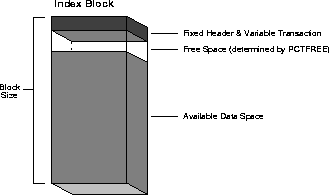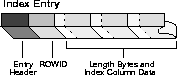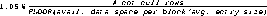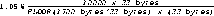# A Space Estimations for Schema Objects

This appendix contains equations that can help you approximate the amount of space for specific schema objects. Constants in estimate calculations are operating system-specific.

 Attention: While these equations help estimate schema object size, they are approximations, and may vary from your actual results.

## Estimating Space Required by Non-Clustered Tables

The procedures in this section describe how to estimate the total number of data blocks necessary to hold data inserted into a non-clustered table. Within this sample calculation, no concurrency is assumed, and users are not performing intervening delete or update operations.

 Note: This is a best-case scenario only when users insert rows without performing deletes or updates.

Typically, the space required to store a set of rows will exceed this calculation when updates and deletes are also being performed on the table. The actual space required for complex workloads is best determined empirically, and then scaled by the number of rows in the table. In general, increasing amounts of concurrent activity on the same data block results in additional overhead (for transaction records), so it is important that you take into account such activity when scaling empirical results.

##### To Calculate Space Required by Non-Clustered Tables

1. Calculate the total block header size.
2. Calculate the available data space per data block.
3. Calculate the space used per row.
4. Calculate the total number of rows that will fit in a data block.

#### Step 1: Calculate the Total Block Header Size

The space required by the data block header is the result of the following formula:

```Space after headers (hsize)
=
DB_BLOCK_SIZE - KCBH - UB4 - KTBBH - ((INITRANS - 1) * KTBIT) - KDBH

```

Where:

 DB_BLOCK_ SIZE is the database block size as viewed in the V\$PARAMETER view KCBH, UB4, KTBBH, KTBIT,KDBH are constants whose sizes you can obtain by selecting from entries in the V\$TYPE_SIZE view INITRANS is the initial number of transaction entries allocated to the table

#### Step 2: Calculate the Available Data Space Per Data Block

The space reserved in each data block for data, as specified by PCTFREE, is calculated as follows:

```available data space (availspace)
=
CEIL(hsize * (1 - PCTFREE/100)) - KDBT

```

Where:

 CEIL rounds a fractional result to the next highest integer PCTFREE is the percentage of space reserved for updates in the table KDBT is a constant whose size you can obtain by selecting the entry from the V\$TYPE_SIZE view

 Note: If you are unable to locate the value of KDBT, use the value of UB4 instead.

#### Step 3: Calculate the Space Used per Row

Calculating the amount of space used per row is a multi-step task.

First, you must calculate the column size, including byte lengths:

```Column size including byte length
=
column size + (1, if column size < 250, else 3)

Note:

You can also determine column size empirically, by selecting avg(vsize(colname)) for each column in the table.

```

Then, calculate the row size:

```

Rowsize
=
row header (3 * UB1) + sum of column sizes including length bytes

```

Finally, you can calculate the space used per row:

```Space used per row (rowspace)
=
MAX(UB1 * 3 + UB4 + SB2, rowsize) + SB2

```

Where:

 UB1, UB4, SB2 are constants whose size can be obtained by selecting entries from the V\$TYPE_SIZE view

When the space per row exceeds the available space per data block, but is less than the available space per data block without any space reserved for updates (for example, available space with PCTFREE=0), each row will be stored in its own block.

When the space per row exceeds the available space per data block without any space reserved for updates, rows inserted into the table will be chained into 2 or more pieces, hence, this storage overhead will be higher.

Figure A-1 depicts elements in a table row.

#### Step 4: Calculate the Total Number of Rows That Will Fit in a Data Block

You can calculate the total number of rows that will fit into a data block using the following equation:

```Number of rows in block
=
FLOOR(availspace / rowspace)

```

Where:

 FLOOR rounds a fractional result to the next lowest integer

In summary, remember that this procedure provides a reasonable estimate of a table's size, not an exact number of blocks or bytes. After you have estimated the size of a table, you can use this information when specifying the INITIAL storage parameter (size of the table's initial extent) in your corresponding CREATE TABLE statement.

See Also: See your operating system-specific Oracle documentation for any substantial deviations from the constants provided in this procedure.

#### Space Requirements for Tables in Use

After a table is created and in use, the space required by the table is usually higher than the estimate derived from your calculations. More space is required due to the method by which Oracle manages free space in the database.

## Estimating Space for Indexes

The calculations in the procedure rely on average column lengths of the columns that constitute an index; therefore, if column lengths in each row of a table are relatively constant with respect to the indexed columns, the estimates calculated by the following procedure are more accurate. Also, the following factors can impact the accuracy of your calculations:

• port-specific variables
• the 5 per cent multiplication factor in the branch blocks portion, which is an arbitrary variable
• internal fragmentation
##### To Estimate Space for Indexes

1. Calculate the total block header size.
2. Calculate the available data space per data block.
3. Calculate the combined column lengths of an average index value.
4. Calculate the total average index value size.
5. Calculate the number of blocks and bytes required for the index.

 Note: Several calculations are required to obtain a final estimate, and several of the constants (indicated by *) provided are operating system-specific. Your estimates should not significantly differ from actual values.

See Also: See your operating system-specific Oracle documentation for any substantial deviations from the constants provided in the following procedure.

#### Step 1: Calculate the Total Block Header Size

Figure A-2 shows the elements of an index block used in the following calculations. The space required by the data block header of a block to contain index data is given by the formula:

```block header size = fixed header + variable transaction header

```

where:

 fixed header 113 bytes variable transaction header 24*I is the value of INITRANS for the index.

If INITRANS =2 (the default for indexes), the previous formula can be simplified:

```block header = 113 + (24*2) bytes
= 161 bytes

Figure A-2  Calculating the Space for an Index```

#### Step 2: Calculate Available Data Space Per Data Block

The space reserved in each data block for index data, as specified by PCTFREE, is calculated as a percentage of the block size minus the block header:

```
```
```available data  = (block size - block header) -
space per block   ((block size - block header)*(PCTFREE/100))

```

The block size of a database is set during database creation and can be determined using the Server Manager command SHOW, if necessary:

```SHOW PARAMETERS db_block_size;

```

If the data block size is 2K and PCTFREE=10 for a given index, the total space for new data in data blocks allocated for the index is:

```available data space per block
= (2048 bytes - 161 bytes) -
((2048 bytes - 161 bytes)*(10/100))
= (1887 bytes) - (1887 bytes * 0.1)
= 1887 bytes - 188.7 bytes
= 1698.3 bytes

```

#### Step 3: Calculate Combined Column Lengths

The space required by the average value of an index must be calculated before you can complete Step 4, calculating the total row size. This step is identical to Step 3 in the procedure for calculating table size, except you only need to calculate the average combined column lengths of the columns in the index.

#### Step 4: Calculate Total Average Index Value Size

Figure A-3 shows elements of an index entry used in the following calculations. Once you have calculated the combined column length of an average index entry, you can calculate the total average entry size according to the following formula:

```bytes/entry = entry header + ROWID length + F + V + D

```

Where:

 entry header 2 bytes ROWID length 6 bytes F Total length bytes of all columns that store 127 bytes or less. The number of length bytes required by each column of this type is 1 byte. V Total length bytes of all columns that store more than 127 bytes. The number of length bytes required by each column of this type is 2 bytes. D Combined data space of all index columns (from Step 3).

#### Figure A-3 Calculating the Average Size of an Index EntryFor example, given that D is calculated to be 22 bytes and that the index is comprised of three VARCHAR(10) columns, the total average entry size of the index is:

```avg. entry size = 2 + 6 + (1 * 3) + (2 * 0) + 22 bytes
= 33 bytes

Note:

For a non-unique index, the ROWID is considered another column, so it must have one length byte.

```

#### Step 5: Calculate Number of Blocks and Bytes

Calculate the number of blocks required to store the index using the following formula:

```# blocks for index =
```Note: The additional 5% added to this result (by means of the multiplication factor of 1.05) accounts for the extra space required for branch blocks of the index.

For example, continuing with the previous example, and assuming you estimate that indexed table will have 10000 rows that contain non-null values in the columns that constitute the index:

```# blocks for index =
```
``````

This results in 204 blocks. The number of bytes can be calculated by multiplying the number of blocks by the data block size.

Remember that this procedure provides a reasonable estimate of an index's size, not an exact number of blocks or bytes. Once you have estimated the size of a index, you can use this information when specifying the INITIAL storage parameter (size of the index's initial extent) in your corresponding CREATE INDEX statement.

#### CreatingTemporary Space Required for Index

When creating an index for a loaded table, temporary segments are created to sort the index. The amount of space required to sort an index varies, but can be up to 110% of the size of the index.

 Note: Temporary space is not required if the NOSORT option is included in the CREATE INDEX command. However, you cannot specify this option when creating a cluster index.

## Estimating Space Required by Clusters

The following procedure demonstrates how to estimate the initial amount of space required by a set of tables in a cluster. This procedure estimates only the initial amount of space required for a cluster. When using these estimates, note that the following items can affect the accuracy of estimations:

• Trailing nulls are not stored, nor is a length byte.
• Inserts of, updates to, and deletes of rows, as well as tables containing columns larger than a single data block can cause fragmentation and chained row pieces. Therefore, the following estimates may tend to be lower that the actual space required if significant fragmentation occurs.

Once you calculate a table's size using the following procedure, you should add about 10 to 20 per cent additional space to calculate the initial extent size for a working table.

##### To Estimate Space Required by Clusters

1. Calculate total block header size and space available for table data.
2. Calculate the combined column lengths of the average rows per cluster key.
3. Calculate the average row size of all clustered tables.
4. Calculate the average cluster block size.
5. Calculate the total number of blocks required for the cluster.

#### Step 1: Calculate Total Block Header Size and Space Available for Table Data

The following formula returns the amount of available space in a block:

 Note: Several calculations are required to obtain a final estimate, and several of the constants (indicated by *) provided are operating system-specific. Your estimates should not significantly differ from actual values. See your operating system-specific Oracle documentation for any substantial deviations from the constants provided in the following procedure.

```space left in block after headers (hspace)
=  BLOCKSIZE - KCBH - UB4 - KTBBH - KTBIT*(INITTRANS - 1) - KDBH

```

where the sizes of KCBH, KTBBH, KTBIT, KDBH, and UB4 can be obtained by selecting * from v\$type_size table.

 Note: If this is a table segment (instead of the cluster segment shown above), the table directory would simply be 4.

Then use the following formula to calculate the space available for table data:

```space available for table data
= hspace*(1 - PCTFREE/100) - 4*(NTABLES + 1) * ROWSINBLOCK

```

Where:

 BLOCKSIZE is the size of a data block INITTRANS is the initial number of transaction entries for the object PCTFREE is the percentage of space to reserve in a block for updates NTABLES is the number of tables in the cluster ROWS INBLOCK is the number of rows in a block

#### Step 2: Calculate Space Required by a Row

Use Step 3 from the procedure in "Estimating Space Required by Non-Clustered Tables" to calculate this number. Make note of the following caveats:

• Calculate the data space required by an average row for each table in the cluster. For example, in a cluster that contains tables T1 and T2, calculate the average row size for both tables.
• Do not include the space required by the cluster key in any of the above calculations. However, make note of the space required to store an average cluster key value for Step 5. For example, calculate the data space required by an average row in table T1, not including the space required to store the cluster key.
• Do not include any space required by the row header (that is, the length bytes for each column); this space is accounted for in the next step.

For example, assume two clustered tables are created with the following statements:

```CREATE TABLE t1 (a CHAR(10), b DATE, c NUMBER(10,2))
CLUSTER t1_t2 (c);

CREATE TABLE t2 (c NUMBER(10,2), d CHAR(10))
CLUSTER t1_t2 (c);

```

Notice that the cluster key is column C in each table.

Considering these example tables, the space required for an average row (D1) of table T1 and the space required for an average row (D2) of table T2 is:

```D1 (space/average row)	  = (a + b)
= (10 + 7) bytes
= 17 bytes

D2 (space/average row)	  = (d)
= 10 bytes

```

#### Step 3 Calculate Total Average Row Size

You can calculate the minimum amount of space required by a row in a clustered table according to the following equation:

```Sn bytes/row = row header + Fn + Vn + Dn

```

Where:

 row header* 4 bytes per row of a clustered table. Fn Total length bytes of all 250 bytes or less. The number of length bytes required by each column of this type is 1 byte. Vn Total length bytes of all columns in table n that store more than 250 bytes. The number of length bytes required by each column of this type is 3 bytes. Dn Combined data space of all columns in table n (from Step 3).

 Note: Do not include the column length for the cluster key in variables F or V for any table in the cluster. This space is accounted for in Step 5.

For example, the total average row size of the clustered tables T1 and T2 are as follows:

```   S1  = (4 + (1 * 2) + (3 * 0) + 17) bytes
= 23 bytes

S    = (4 + (1 * 1) + (3 * 0) + 10) bytes
= 15 bytes

Note:

The absolute minimum row size of a clustered row is 10 bytes, and is operating system-specific. Therefore, if your calculated value for a table's total average row size is less than these absolute minimum row sizes, use the minimum value as the average row size in subsequent calculations.

```

#### Step 4: Calculate Average Cluster Block Size

To calculate the average cluster block size, first estimate the average number of rows (for all tables) per cluster key. Once this is known, use the following formula to calculate average cluster block size:

```avg. cluster block size (bytes)=
((R1*S1) + (R2*S2) + .. + (Rn*Sn)) + key header + Ck + Sk + 2Rt

```

Where:

 Rn The average number of rows in table n associated with a cluster key. Sn The average row size in table n (see Step 4). key header* 19 Ck Column length for the cluster key. Sk Space required to store average cluster key value. Rt Total number of rows associated with an average cluster key (R1 + R2 ... + Rn). This accounts for the space required in the data block header for each row in the block.

For example, consider the cluster that contains tables T1 and T2. An average cluster key has one row per table T1 and 20 rows per table T2. Also, the cluster key is of datatype NUMBER (column length is 1 byte), and the average number is 4 digits (3 bytes). Considering this information and the previous results, the average cluster key size is:

```SIZE = ((1 * 23) + (20 * 15) + 19 + 1 + 3 + (2 * 21)) bytes
= 388 bytes

```

Specify the estimated SIZE in the SIZE option when you create the cluster with the CREATE CLUSTER command. This specifies the space required to hold an average cluster key and its associated rows; Oracle uses the value of SIZE to limit the number of cluster keys that can be assigned to any given data block. After estimating an average cluster key SIZE, choose a SIZE somewhat larger than the average expected size to account for the space required for cluster keys on the high side of the estimate.

To estimate the number of cluster keys that will fit in a database block, use the following formula, which uses the value you calculated in Step 2 for available data space, the number of rows associated with an average cluster key (Rt), and SIZE:

```# cluster keys per block
= FLOOR(available data space + 2R / SIZE + 2Rt)

```

For example, with SIZE previously calculated as 400 bytes (calculated as 388 earlier in this step and rounded up), Rt estimated at 21, and available space per data block (from Step 2) calculated as 1742 - 2R bytes, the result is as follows:

```# cluster keys per block
= FLOOR((1936 - 2R + 2R) / (400 + 2 * 21))

= FLOOR(1936 / 442)
= FLOOR(4.4)
= 4

```

#### Step 5: Calculate Total Number of Blocks

To calculate the total number of blocks for the cluster, you must estimate the number of cluster keys in the cluster. Once this is estimated, use the following formula to calculate the total number of blocks required for the cluster:

```# blocks = CEIL(# cluster keys / # cluster keys per block)

Note:

If you have a test database, you can use statistics generated by the ANALYZE command to determine the number of key values in a cluster key. See "Analyzing Tables, Indexes, and Clusters" on page  -3.

```

For example, assume that there are approximately 500 cluster keys in the T1_T2 cluster:

```# blocks T1_T2 = CEIL(500/3)
= CEIL(166.7)
= 167

```

To convert the number of blocks to bytes, multiply the number of blocks by the data block size.

This procedure provides a reasonable estimation of a cluster's size, but not an exact number of blocks or bytes. Once you have estimated the space for a cluster, you can use this information when specifying the INITIAL storage parameter (size of the cluster's initial extent) in your corresponding CREATE CLUSTER statement.

#### Space Requirements for Clustered Tables in Use

Once clustered tables are created and in use, the space required by the tables is usually higher than the estimate given by the previous section. More space is required due to the method Oracle uses to manage free space in the database.

## Estimating Space Required by Hash Clusters

As with index clusters, it is important to estimate the storage required for the data in a hash cluster. Use the procedure described in "Estimating Space Required by Clusters" on page -10, with the following additional notes:

• A sub-goal of the procedure is to determine the SIZE of each cluster key. However, for hash clusters, the corresponding sub-goal is to determine the SIZE of each hash key. Therefore, you must consider not only the number of rows per cluster key value, but also the distribution of cluster keys over the hash keys in the cluster.
• In Step 3, make sure to include the space required by the cluster key value. Unlike an index cluster, the cluster key value is stored with each row placed in a hash cluster.
• In Step 5, you are calculating the average hash key size, not cluster key size. Therefore, take into account how many cluster keys map to each hash value. Also, disregard the addition of the space required by the cluster key value, Ck. This value has already been accounted for in Step 3 (see previous item).